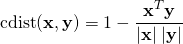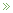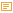• ## Float Precision

Posted by Konstantin 13.01.2015 No Comments

I haven't updated this blog for quite some time, which is a shame. Before I resume I wanted to reproduce here a couple of my old posts from other places. This particular piece stems from a post on our research group's webpage from more than 8 years ago, but is about an issue that never stops popping up in practice.

Precision of floating point numbers is a very subtle issue. It creeps up so rarely that many people (me included) would get it out of their heads completely before stumbling upon it in some unexpected place again.

Indeed, most of the time it is not a problem at all, that floating point computations are not ideally precise, and no one cares about the small additive noise that it produces, as long as you remember to avoid exact comparisons between floats. Sometimes, however, the noise can severely spoil your day by violating the core assumptions, such as "distance is always greater than zero", or "cosine of an angle never exceeds 1".

The following is, I think, a marvelous example, discovered by Alex, while debugging an obscure problem in one Python program. The choice of the language is absolutely irrelevant, however, so I took the liberty of presenting it here using Javascript (because this lets you reproduce it in your browser's console, if you wish). For Python fans, there is an interactive version available here as well.

A cosine distance metric is a measure of dissimilarity of two vectors, often used in information retrieval and clustering, that is defined as follows:A straightforward way to put this definition into code is, for example, the following:

function length(x) {
return Math.sqrt(x*x + x*x);
}

function cosine_similarity(x, y) {
return (x*y + x*y)/length(x)/length(y);
}

function cosine_distance(x, y) {
return 1 - cosine_similarity(x, y);
}

Now, mathematically, the cosine distance is a valid distance function and is thus always positive. Unfortunately, the floating-point implementation of it presented above, is not the same. Check this out:

> Math.sign(cosine_distance([6.0, 6.0], [9.0, 9.0]))
< -1

Please, beware of float comparisons. In particular, think twice next time you use the sign() function.

Tags: , ,

### Calendar

January 2015
M T W T F S S
« Mar   Mar »
1234
567891011
12131415161718
19202122232425
262728293031